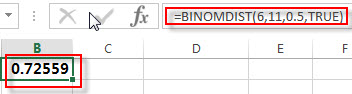## Excel BINOMDIST Function

This post will guide you how to use the BINOMDIST function with syntax and examples in Microsoft excel.

### Description

The Excel BINOMDIST function returns the individual term binomial distribution probability. For example, you can use the BINOMDIST function to calculate the probability that two of the next three babies born are male in Excel. And this function has been replaced with another new function BINOM.DIST.

The BINOMDIST function is a build-in function in Microsoft Excel and it is categorized as a Statistical Function.

The BINOMDIST function is available in Excel 2016, Excel 2013, Excel 2010, Excel 2007, Excel 2003, Excel XP, Excel 2000, Excel 2011 for Mac.

### Syntax

The syntax of the BINOMDIST function is as below:

`= BINOMDIST (number_s, trials, probability_s, cumulative)`

Where the BINOMDIST function arguments are:

• number_s -This is a required argument. The number of successes that you want to calculate the probability.
• Trials – This is a required argument.  The number of independent trials.
• probability_s – This is a required argument.  The probability of success for each trial.
• Cumulative – This is a required argument. It is a logical value that specifies the type of binomial distribution to be used. If the cumulative is TRUE, the BINOMDIST function returns the cumulative distribution function; if it is equal to FALSE, the BINOMDIST function returns the probability mass function.

Note:

• If number_s, trials, or probability_s argument is non-numeric, the BINOMDIST function will return #VALUE! Error.
• If number_s and trials arguments are not integers and the BINOMDIST function will truncate it.
• If number_s argument is smaller than 0 or greater than trials argument, the BINOMDIST function will return the #NUM! Error.
• If probability_s is small than 0 or greater than 1, then the BINOMDIST function will return the #NUM! Error.

### Excel BINOMDIST Function Examples

The below examples will show you how to use Excel BINOMDIST Function to get the binomial distribution probability for a given number of successes.

Example 1: to calculate the individual term binomial distribution probability for a given number of successes from a specified number of trials, using the following formula:

=BINOMDIST(6,11,0.5,TRUE)### Related Functions

• Excel BINOM.DIST function
The Excel BINOM.DIST function returns the individual term binomial distribution probability. The syntax of the BINOM.DIST function is as below:= BINOM.DIST(number_s, trials, probability_s, cumulative).…
• Excel BETA.DIST function
The Excel BETA.DIST function can be used to calculate the cumulative beta distribution or beta probability density function.The syntax of the BETA.DIST function is as below:= BETA.DIST(x, alpha, beta, cumulative, [A], [B]).…